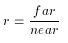# glFrustum

### NAME

glFrustum -- multiply the current matrix by a perspective matrix

### C SPECIFICATION

void glFrustum(GLdouble left,
GLdouble right,
GLdouble bottom,
GLdouble top,
GLdouble near,
GLdouble far)


### PARAMETERS

left, right
Specify the coordinates for the left and right vertical clipping planes.

bottom, top
Specify the coordinates for the bottom and top horizontal clipping planes.

near, far
Specify the distances to the near and far depth clipping planes. Both distances must be positive.

### DESCRIPTION

glFrustum describes a perspective matrix that produces a perspective projection. (left,bottom,-near) and (right,top,-near) specify the points on the near clipping plane that are mapped to the lower left and upper right corners of the window, respectively, assuming that the eye is located at (0, 0, 0). -far specifies the location of the far clipping plane. Both near and far must be positive. The corresponding matrix isThe current matrix is multiplied by this matrix with the result replacing the current matrix. That is, if M is the current matrix and F is the frustum perspective matrix, then M is replaced with M*F.

Use glPushMatrix and glPopMatrix to save and restore the current matrix stack.

### NOTES

Depth buffer precision is affected by the values specified for near and far. The greater the ratio of far to near is, the less effective the depth buffer will be at distinguishing between surfaces that are near each other. Ifroughly ld(r) bits of depth buffer precision are lost. Because r approaches infinity as near approaches zero, near must never be set to zero.

### ERRORS

GL_INVALID_VALUE is generated if either near or far is not positive.

GL_INVALID_OPERATION is generated if glFrustum is called between a call to glBegin and the corresponding call to glEnd.

### ASSOCIATED GETS

glGet with argument GL_MATRIX_MODE
glGet with argument GL_MODELVIEW_MATRIX
glGet with argument GL_PROJECTION_MATRIX
glGet with argument GL_TEXTURE_MATRIX